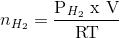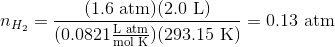# College Chemistry : Partial Pressure

## Example Questions

### Example Question #1 : Partial Pressure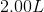mixture of helium, nitrogen, and neon has a total pressure of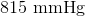at a temperature of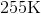. If the partial pressure of helium is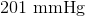and the partial pressure of nitrogen is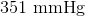, what mass of neon is present in the mixture?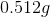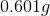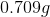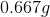Explanation:

Since we have the total pressure and the pressures of helium and nitrogen, we can find the pressure of neon in this container.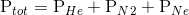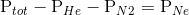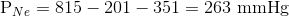Next, convert this pressure into atmospheres.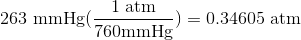Now, recall the Ideal Gas Law: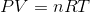Rearrange the equation to solve for moles of gas: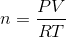Using the pressure of neon, find the moles of neon that is present in the container.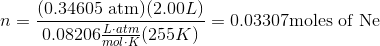Finally, convert the moles of neon into grams of neon using the molar mass.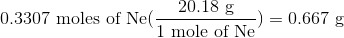Make sure your answer hassignificant digits.

### Example Question #2 : Partial Pressure

Suppose that hydrochloric acid and aluminum are reacted to produce hydrogen gas. This hydrogen gas is collected by displacement of water at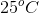and a total pressure of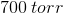. If the volume of the gas collected is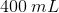, then how many moles of hydrogen were collected?

Note: The vapor pressure of water atis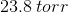.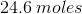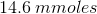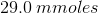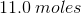Explanation:

For this question, we're told that a chemical reaction is producing hydrogen gas. In doing so, the gas is displacing a certain amount of water vapor. We're asked to determine the number of moles of gas produced in this process.

The amount of water displaced by the production of hydrogen gas will be the amount (volume) of hydrogen gas produced. To measure its pressure, we take the reading of the total pressure and then subtract from it the pressure of water vapor (since it contributes to the total pressure). Using this information, we can use the ideal gas equation to determine the number of moles of hydrogen gas.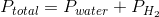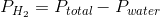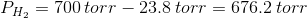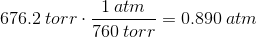Next, we can use the ideal gas equation to solve for our answer.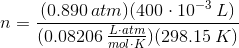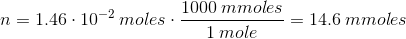### Example Question #3 : Partial Pressure

A 4.0 L container at 25 C is filled with 2.0 g neon and 8.0 g helium. What is the total pressure of the mixture?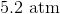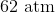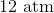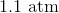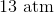Explanation:

To start, first recognize that this is an ideal gas law problem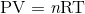Rearranging the equation to solve for P gives us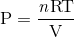To solve the problem, we now need to find the total number of moles in the gaseous mixture ntot. To do this, convert the values for Ne and He to moles and add them together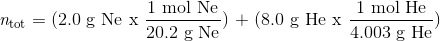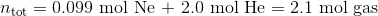Now that we have ntot, plug known values into the ideal gas law equation. Remember to convert from Celsius to Kelvin!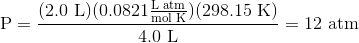### Example Question #4 : Partial Pressure

A 2.0 L container at 20 oC contains a gaseous mixture of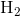and He with a total pressure of 4 atm. The partial pressure exerted by He is 2.4 atm. How many moles ofare in the container?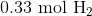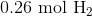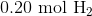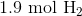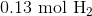Explanation:

This is another ideal gas law problem, but this time dealing with partial pressures. Total pressure of a mixture is the sum of the partial pressures of each component. So first calculate the partial pressure exerted by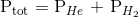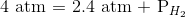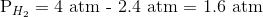Now that we have solved for the partial pressure of, we can solve for moles ofby using the ideal gas law equation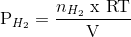Rearrange to solve for moles of